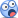# Lognormal

#### mariosantos

##### New Member
Subscriber
Dear community,

I've got a question about what drift to use when calculating the expected future value of a firm assets under the assumption that its assets follow a GBM.

In this paper it is quite clear that it is Mu + Sigma^2*0,5 the number that should be used (see last page):

http://www.columbia.edu/~ks20/FE-Notes/4700-07-Notes-GBM.pdf

But David uses Mu - Sigma^2*0,5 in Servigny study notes.

¿Why is this happening?

#### ShaktiRathore

##### Well-Known Member
Subscriber
Hi
David is right when he talks about the drift of returns. The drift component should be Mu - 0.5*Sigma^2 for returns of the stock.But the above paper i think simply assumes the drift component as Mu +0.5*Sigma^2 instead of Mu - 0.5*Sigma^2 .Thereafter the paper correctly considers the term Mu - 0.5*Sigma^2 as risk neutral drift.
Yes technically the correct drift term is Mu - 0.5*Sigma^2 only.The paper assumed that drift is Mu +0.5*Sigma^2 i think.
thanks

Last edited:

#### jairamjana

##### Member
I think you are talking about Merton model for computing probability of default.

For the short answer we use Mu - Sigma^2*0,5 because this is similar to d2 of Black-Scholes option pricing model..
This is how you use in excel..
NORM.S.DIST((-(LN((V(0)*EXP(mu-sigma^2)/2)*SQRT(T-t)/K)/sigma))/sigma*SQRT(T-t)),TRUE)

And this Black Scholes d2 which looks like this.Of course you might not be familiar with this way of presenting but rather this is more familiar and more elegant..But rest assured both are same#### jairamjana

##### Member
@David Harper CFA FRM I understood the application of the Merton Model but in your example you usedV(0) as 12.75$.. I haven't read De Servigny's book Measuring and managing Credit Risk but in GARP Material V(0) is 12.511$((LN((12.511*EXP(5%-((9.6%)^2)/2))/10))/9.6%) = 2.8064

and​

NORM.S.DIST((-(LN((12.511*EXP(5%-((9.6%)^2)/2))/10))/9.6%),TRUE) = 0.25%
Time variable T-t is ignored because its 1 year.
Because you have taken 12.75$and not 12.511$ the PD is halved.. 0.25% in GARP as against 0.13% in BT#### brian.field

##### Well-Known Member
Subscriber
I think we can simplify this a bit - the expected value of a lognormal random variable Y=e^X, or E(Y) is e^(m+0.5v^2) where X is distributed as N(m,v^2).

#### ShaktiRathore

##### Well-Known Member
Subscriber
I think we can simplify this a bit - the expected value of a lognormal random variable Y=e^X, or E(Y) is e^(m+0.5v^2) where X is distributed as N(m,v^2).
So lets settle for that Mu +0.5*Sigma^2 is the drift of lognormal random variable as discussed in the paper.And Mu-0.5*Sigma^2 is risk neutral drift that we often comes across in the Black scholes(BSM) derivation where we assume a risk free world.
thanks

#### David Harper CFA FRM

##### David Harper CFA FRM
Staff member
Subscriber
The paper seems to support, not contradict. The relevant BSM equation, i think, is (formula #22 on page 6) the risk-neutral drift, u* = r - σ^2/2. The Merton replaces this (r) with asset return, such that BSM's N(d2)--i.e., with the risk-free rate--is the risk-neutral probability of option exercise; switch the risk free rate with asset return and N(-d2) becomes the "real-word" probability the company will default under Merton. In my opinion, the safest "memory shortcut" per my linked note (thank you @ShaktiRathore ) is d2 in BSM. N(d2) in BSM is risk neutral probability of ITM/excerise; N(-d2), with asset return replacing risk free rate, Merton PD. Same dynamics. Also, page (7) of the paper supports this, although notice he shows it both ways (eg, de servigny shows it the opposite of Stulz, but it's the same formula, these returns are normal so you can go either direction, so to speak): -[ln(K/S) - (r - σ^2/2)T]/[σ*sqrt(T)] = +[ln(S/K) + (r - σ^2/2)T]/[σ*sqrt(T)].

There is a good question about how the subtracted σ^2/s appears, which Hull briefly mentions in his The Black-Scholes-Merton chapter per the lognormal property but I have always thought McDonald explains it best (screenshot, sorry) in his (McDonald's Chapter 18 The Lognormal Distribution, Derivatives Markets, 3rd Ed)#### mariosantos

##### New Member
Subscriber
I think we can simplify this a bit - the expected value of a lognormal random variable Y=e^X, or E(Y) is e^(m+0.5v^2) where X is distributed as N(m,v^2).
Thanks!

#### mariosantos

##### New Member
Subscriber
Thanks to everybody for your extensive help. It is awesome how helpful this community is, I hope I could correspond you in the future.

Kind regards.